Impedance and Impedance Matching

Overview

This tutorial recommends tips and techniques for using National Instruments high-speed digitizers to build the most effective data sampling system possible. In this tutorial, you will learn fundamental information about the underlying theory of sampling with a high-speed digitizer and various methods to optimize the performance of your data sampling. This section of the tutorial covers the topics below.

What is Impedance?

Impedance is defined as the combined effect of capacitance, inductance, and resistance that a circuit offers a signal at a given frequency. Impedance can also be defined as a measure of resistance to electrical current flow when a voltage is moved across it. Impedance is measured in ohms and is the ratio of voltage to the flow of current allowed.

Input impedance is measured across the input terminals of a circuit. In relation to a high-speed digitizer, input impedance is usually defined as the effective resistance and capacitance seen at the input to the digitizer. The impedance can vary when the digitizer is powered on, powered off, and when isolated input limits are overloaded. In general, the higher the input impedance of the digitizer, the less the digitizer will disturb the signal being measured.

Signal Reflection

Signal reflection is conceptually similar to the behavior of waves as they pass between mediums of different densities.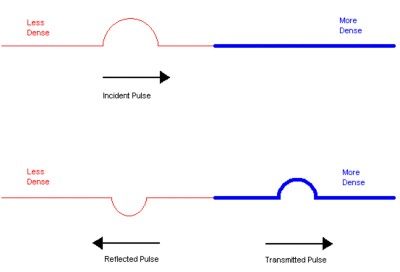Figure 1. Pulse wave reflection and transmission between mediums of different densities

Similarly, signal reflection occurs as a transmitted signal is reflected back toward its origin due to differences in impedance along the transmission line.

Impedance Matching

When high-frequency signals are carried on transmission lines of any significant length, care must be taken that the transmission medium is matched to its terminations. The source and load impedances should equal the characteristic impedance of the transmission line, as this minimizes signal reflections. The presence of impedance discontinuities or mismatches will degrade the amplitude and phase accuracy, as well as the temporal fidelity, of measurements made with a digitizer. The example below shows one of the most common mismatch errors encountered in such measurements.

Example

Selectable termination impedances are provided at the digitizer inputs to accommodate the most popular coaxial cable characteristic impedances: 50 Ω and 75 Ω. The diagram below illustrates what happens when a coaxial cable of the wrong characteristic impedance (75 Ω) is used with 50 Ω source and load impedances.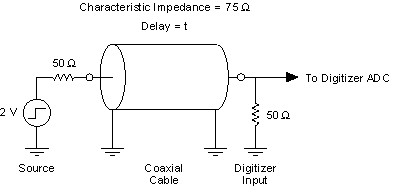Figure 2. Cable with characteristic impedance of 75 Ω connected to load and source impedances of 50 Ω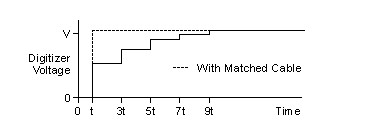Figure 3. Distortion resulting from a cable's impedance mismatched with the impedance of a source and load

The pulse encounters impedance mismatches at each end of the cable, whereupon it is partially reflected. The reflected pulse traverses the cable back and forth numerous times, diminishing at each end by the reflection coefficient,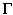.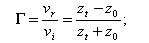where

vr = reflected voltage
vi
= incident voltage
zt
= termination impedance
z0
= characteristic impedance

The resulting voltage waveform recorded by the digitizer is distorted by the asymptotic decay of the reflected pulse, as shown above in Figure 3, exaggerated for visual effect. Impedance discontinuities of smaller magnitude and/or duration have correspondingly smaller effects. Also displayed is the waveform that results when a cable of matched impedance (50 Ω) is used.

Mismatch Uncertainty

Impedance matching is also important for preserving the absolute power measurement accuracy of a digitizer. The accuracy with which power can be measured is limited by mismatch error. The mismatch error in a system can be shown to be bounded by: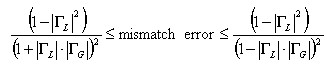whereL = load reflection coefficientG = generator reflection coefficient

The denominator term represents mismatch uncertainty, which is a fundamental limit to the power transfer accuracy that can be achieved across a mismatched junction.

Resistive Matching

Signal sources with low (high) source impedance can be matched with a resistor placed in series (shunt) such that the total source impedance (admittance) is matched to the cable characteristic impedance (admittance). Sources that are not capable of driving the cable impedance directly can be coupled through a matching impedance pad or L-pad (a basic network structure: one shunt and one series branch, which gives an arrangement in the shape of an L). An example of an L-pad is shown in Figure 4. In this case, the source sees a 500 Ω load, while the source impedance presented to the cable is 50 Ω. High-frequency components and layout techniques should be used throughout to minimize parasitic effects.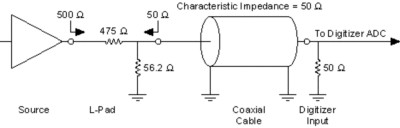Figure 4. Example of an L-pad

Additionally, impedance matching pads can be purchased through component manufacturers in common form factors (such as a BNC connector). These impedance matching pads are designed to take some input impedance (for example, 75 Ω) and produce an output impedance (for example, 50 Ω). These pads are generally inexpensive and easy to use.